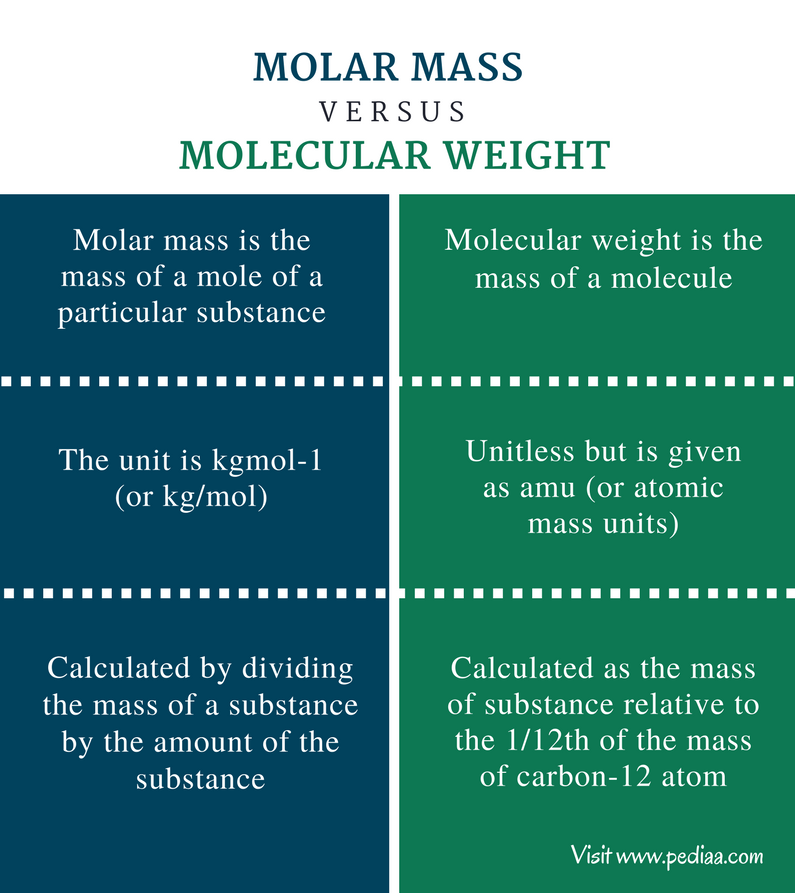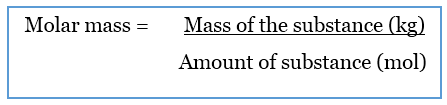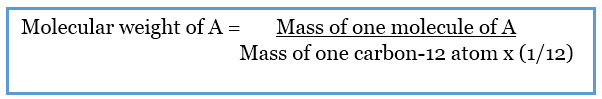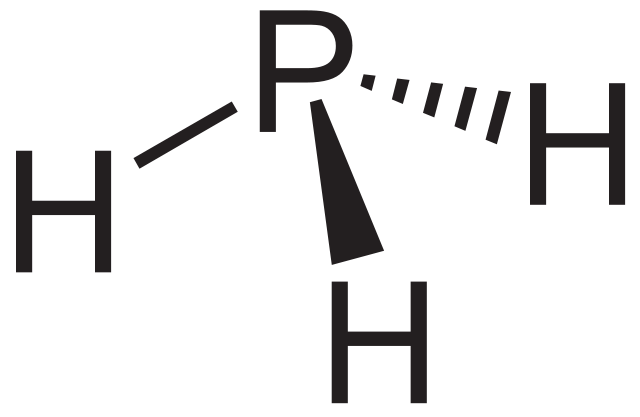Difference Between Molar Mass and Molecular Weight

Main Difference – Molar Mass vs Molecular Weight

Molecules are substances formed due to combinations of same or different atoms in different ratios. These molecules can get attached to each other to form compounds. Almost all chemical reactions are involved the changes occur in molecules or compounds. Therefore, it is very important to understand the chemical and physical properties of molecules in order to conduct chemical reactions and predict the final products. Molar mass and molecular weight are two such physical properties of substances. The main difference between molar mass and molecular weight is that molar mass gives the mass of a mole of a particular substance whereas molecular weight is the mass of a molecule of a particular substance.

Key Areas Covered

1. What is Molar Mass
– Definition, Formula, Units, Calculation
2. What is Molecular Weight
– Definition, Formula, Units, Calculation
3. What is the difference between Molar Mass And Molecular Weight
– Comparison of Key Differences

Key Terms: Atoms, Carbon, Isotope, Molar Mass, Mole, Molecular WeightWhat is Molar Mass

Molar mass is the mass of a mole of a particular substance. It is a physical property of substances. The molar mass is obtained by dividing the mass of a particular substance by the amount of it.The SI unit for molar mass is kgmol-1 or kg/mol. But in general, it is given in the unit gmol-1 or g/mol.

The molar mass of an element is the atomic mass of that element. Therefore, the molar mass of a substance can be calculated by adding the atomic masses of each element present in that molecule.

Calculation of Molar Mass

Let’s calculate the molar mass of H2O compound.

H2O:

• Number of hydrogen atoms present   = 2
• Atomic mass of hydrogen                      = 1.00794 g/mol
• Number of oxygen atoms present        = 1
• Atomic mass of oxygen                           = 15.999 g/mol
• Therefore, the molar mass of H2O       = (2 x 1.00794 g/mol) + (1 x 15.999 g/mol)

= 18.01488 g/mol

This simple calculation indicates that the mass of one mole of H2O compound is 18 g.

What is Molecular Weight

The term molecular weight can be defined as the mass of a molecule. It is also called the relative molecular mass. That is because molecular weight is calculated as the mass, relative to the carbon-12 isotope.

Considering a hypothetical molecule called A,The above equation indicates that the molecular weight has no units; this is because the division is done between two masses which has the same units. Therefore, the molecular weight is given as atomic mass units or amu. Using the above equation, we can find the molecular weight of an element or a compound. Therefore, as same as in molar mass, the molecular weight of a particular molecule is equal to the sum of the atomic masses of each element.

Calculation of Molecular Weight

If we consider the same example H2O,

• The number of hydrogen atoms present          = 2
• The number of oxygen atoms present              = 1
• Mass of a hydrogen atom                                    = 1.00794 amu
• Mass of an oxygen atom                                      = 15.999 amu
• Therefore the molecular weight of H2O           = (2 x 1.00794 amu) + (1 x 15.999 amu)

= 18.01488 amu

This indicates that both molar mass and molecular mass are same in their values but are different in their units.Figure 1: Phosphine. The molar mass of phosphine is 33.99758 g/mol. The molecular weight is 33.99758 amu.

Difference Between Molar Mass and Molecular Weight

Definition

Molar Mass: Molar mass is the mass of a mole of a particular substance.

Molecular Weight: The term molecular weight can be defined as the mass of a molecule.

Units

Molar Mass: The unit of molar mass is kgmol-1 (or kg/mol).

Molecular Weight: The molecular weight is unitless but is given as amu (or atomic mass units).

Calculation

Molar Mass: Molar mass is calculated by dividing the mass of a substance by the amount of the substance.

Molecular Weight: Molecular weight is calculated as the mass of substance relative to the 1/12th of the mass of carbon-12 atom.

Conclusion

The main difference between molar mass and molecular weight is that molar mass gives the mass of a mole of a particular substance whereas molecular weight is the mass of a molecule of a particular substance. Although the definition and units are different for molar mass and molecular weight, the value is the same. They are physical properties of substances and are very useful in chemical analysis techniques.

References:

1. Helmenstine, Anne Marie. “What Is Molecular Weight? Chemistry Definition.” ThoughtCo. N.p., n.d. Web. Available here. 21 June 2017.
2. “Why Is Molar Mass Important?” Sciencing. N.p., n.d. Web. Available here. 21 June 2017.

Image Courtesy:

1.”Monophosphan” By NEUROtiker – Own work, Public Domain) via Commons Wikimedia